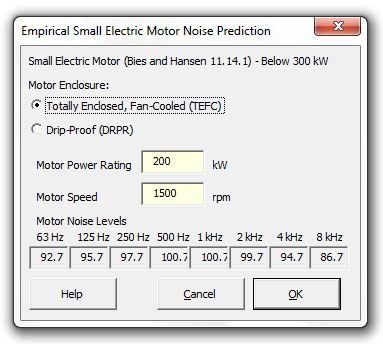### Strutt Help

Empirical Small Electric Motor Noise Prediction    1/1

Strutt|Input Data|Empirical|Electric Motor (Small) inserts sound power levels into the active row of the worksheet.The overall sound pressure level at 1 m has been calculated using the formulae:

Under 40 kW
L_p = 17 + 17log_10(kW) + 15log_10(RPM)

Over 40 kW
L_p = 28 + 10log_10(kW) + 15log_10(RPM)

The octave band sound pressure levels are then calculated by applying the corrections for totally enclosed, fan cooled (TEFC) motor or drip proof (DRPR) motor from Table 11.24 (Bies and Hansen).
Sound power levels have then been calculated by distance correcting to a point source with hemispherical directivity.

References:

• Bies, D., Hansen, C., Engineering Noise Control, 3rd Edition, pp553-554